# User Defined Functions

## UDF procedures

A User Defined function (UDF) is a custom function procedure designed to be called from an Excel worksheet. By default UDF's are listed in the User Defined category of the Insert Function dialog box (figure 0). For information about adding a UDF to a different category see Link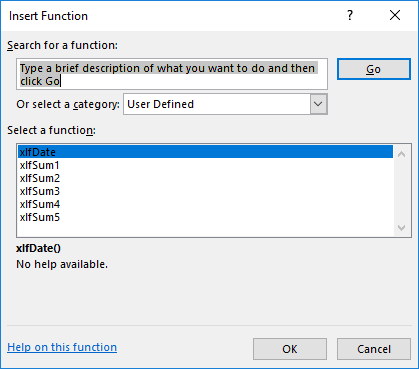Fig 0 Insert Function dialog box showing a list of the User Defined category

Some features of UDF procedures:

• General VBA naming conventions apply except that the name of a UDF must not be a valid cell address. For example, Tax2016 would return a #REF! error in Excel
• Excel naming conventions display all Excel built-in functions in uppercase, such as: SUM, AVERAGE, NPV etc - use a mixture of upper and lower case to distinguish UDFs from Excel's built-in functions
• Remember that functions are designed to only return a "return value", and should not manipulate the Workbook Object or display a message box
• Avoid using the names of Excel built-in functions for UDF procedures. If there is a name clash, include the module name: xlf.sum(), for a sum function in the xlf module
• Functions can return the value with a format (currency, percentage or date and time), in the spirit of NOW , NPV , or IRR , etc

Five examples are shown in the following sections. 1. No arguments, 2. a fixed number of arguments, 3. fixed arguments with multi cell array, 4. optional arguments, and 5. an indeterminate number of arguments.

## 1. UDF with no arguments

A simple function xlfDate() that returns a date in date format.

Code 1: Function procedure xlfDate returns the current date (volatile)
Public Function xlfDate() As String
' Returns the current date in date format (d/m/yy)
Application.Volatile
xlfDate = Format(Date, "General Date")
End Function



Code 1 by line number:

1. Line 1: Procedure declaration: a Public Function procedure named xlfDate with mixed case name. No arguments - the parentheses () are empty. All function procedures in a Code module are Public by default. Line 1 could be written as:
2. Function xlfDate() As String
3. Line 3: Invokes the Application.Volatile method. This means the function will update (recalculate) whenever the worksheet is changed or recalculated (is volatile / changing). This may require change to Manual Workbook Calculation mode to maintain CPU performance. .
4. Line 4: Returns the date in General Date format (d/m/yyyy).

The xlfDate Function Arguments is shown in figure 1.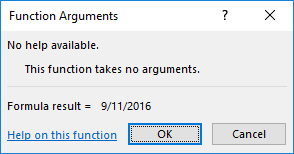Fig 1 Function Arguments dialog box for the xlfDate function - with no arguments

## 2. UDF with a fixed number of arguments

In code 2, the xlfSum1() function (line 11 to line 14) has three compulsory arguments.

Code 2: Function procedure xlfSum1 returns sum of 3 numbers (as cell references in this example)
Sub Run_xlfSum1()
Dim Ans As Double

With ActiveSheet
.Range("C14").Value = 2
.Range("C15").Value = 3
.Range("C16").Value = 4
End With

Ans = xlfSum1([C14], [C15], [C16])
MsgBox "The sum of 2,3, and 4 equals " & Ans, Title:="xlfSum2 demo"

End Sub


Code 2 by line number:

1. Function - line 10 to line 13:
2. Line 10: Procedure declaration: a Public Function procedure named xlfSum1 with mixed case name. Three compulsory arguments - Num1, Num2, and Num3 all of type Double. Each parameter must be a number, or a reference to a single cell. This means that there is no return value until all arguments have valid values.
3. Line 12: Returns the sum of the three arguments
4. Line 13: End of function
5. Run macro - line 15 to line 27:
6. Line 24: could be written as:
7. Ans = xlfSum1(Range("C14"), Range("C15"), Range("C16"))

Ans = xlfSum1(Range("Sheet1!C14"), Range("Sheet1!C15"), Range("Sheet1!C16"))

Ans = xlfSum1(Worksheets("Sheet1").Range("C14"), Worksheets("Sheet1").Range("C15"), Worksheets("Sheet1").Range("C16"))

8. or ...

9. Ans = xlfSum1(2, 3, 4)  ' Using Numbers rather then References

10.To display the Function dialog box from VBA - use the xlDialogFunctionWizard (line 200) or the Range.FunctionWizard method (line 202)

Application.Dialogs(xlDialogFunctionWizard).Show

Activecell.FunctionWizard


### Function Arguments - fundamentals

Here's how the code 2 xlfSum1() function appears in the Function Arguments dialog box (figure 2).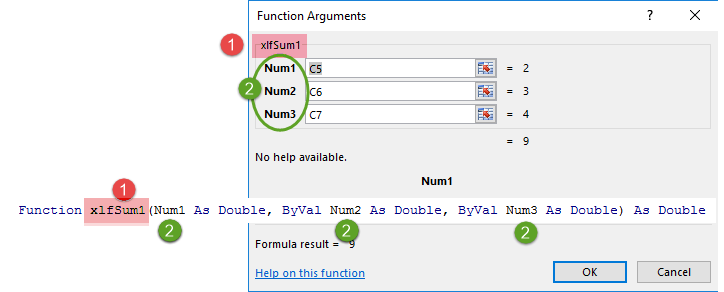Fig 2 Function Arguments for a UDF with compulsory arguments. All displayed in bold font. Inset: declaration line for the xlfSum1 function.

In figure 2:

• the function name: xlfSum1
• the argument names: Num1, Num2, and Num3. All are compulsory so they automatically appear in bold font in the Dialog Box

## 3. UDF with a fixed argument (multi cell range)

### 3.1 Contiguous range

This is another version of the xlfSum function, using a (multi cell) range argument instead of a fixed number of arguments. The range is contiguous, in that, it is one block of data with rectangular shape.

Code 3: Function procedure xlfSum3 returns sum of numbers in a multi cell range
Function xlfSum3(Num As Range) As Double
' Returns the sum of range object
Dim i As Integer
Dim j As Integer

For i = 0 To Num.Rows.Count - 1
For j = 0 To Num.Columns.Count - 1
xlfSum3 = xlfSum3 + Num.Range("A1").Offset(i, j).Value
Next j
Next i

End Function

' ================================
Sub Run_xlfSum3()
Dim Arr As String
Dim Ans As Double

Arr = "={10,20,30,40;"
Arr = Arr & "55,66,77,88;"
Arr = Arr & "90,100,110,120}"

With ActiveSheet
.Range("C35:F37").FormulaArray = Arr
End With

Ans = xlfSum3(Range("C35:F37"))
MsgBox "The sum of range with the array formula equals " & Ans, Title:="xlfSum3 demo"

End Sub


Code 3 by line number:

1. Function - line 60 to line 71:
2. Line 60: Procedure declaration: a Public Function procedure named xlfSum3 with mixed case name. One compulsory argument - Num of type Range. There is no need to use the Set statement when assigning the Range object to the argument name
3. Line 65 to line 69: is a double For...Next loop, reading each element of the Range on a row by row basis
4. Line 67: Processes the cumulative sum of the elements of the Range array
5. Run macro - line 73 to line 88:
6. The macro writes a range of test data to Excel using the VBA version of an Excel array formula (CSE formula)
7. Lines 77 to line 79: Setup the text string for the data array constant, 3 rows by 4 columns
8. Line 77: could be written as:
9. Arr = "={10,20,30,40;55,66,77,88;90,100,110,120}"

10. where the comma (,) separates the columns, and the semi-colon (;) is used to indicate the end of row, except the last row

11. Line 82: Writes the array constant (dimension 3 x 4) as an array formula to the Excel range. Each cell of the range contains the formula {={10,20,30,40;55,66,77,88;90,100,110,120}} (Ready mode)

### 3.2 Noncontiguous range

The function xlfSum3() takes a Range as its only argument. The incorporation of a Range operator allows multiple ranges to be specified.

Range operators:

• Union operator: a comma character "," that combines two or more ranges into a single range reference. Shown in figure 3.1. Wrap the union in brackets () to distinguish the union operator from an argument separator
• Intersection operator: a space character " " that returns the area of overlap (intersection) of multiple Ranges, usually two. Shown in figure 3.2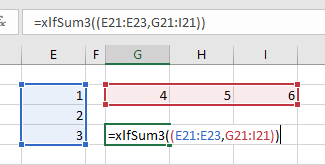Fig 3.1 Function edit mode - a single range formed from the union of two ranges (shown in red and blue). In this case the xlfSum3 returns 21. Note the double brackets ((Num))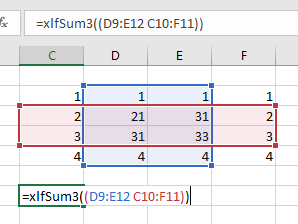Fig 3.2 Function edit mode a single range formed from the intersection of two ranges (the area of overlap of the of the red and blue arrays). In this case the xlfSum3 returns 116 (21 + 31 + 31 + 33). Note the double brackets ((Num))

## 4. UDF with a fixed and optional arguments

In this version (xlfSum4()) there are 2 compulsory arguments and 1 optional argument.

Optional arguments:

• are always listed after compulsory arguments
• are identified by the Optional keyword
• in a UDF are always of the type Variant
• arguments always have a default value, and in a UDF this is handled by the VBA IsMissing function

Code 4: Function procedure xlfSum4 returns sum of 3 numbers (two compulsory and one optional in this example)
Function xlfSum4(Num1 As Double, Num2 As Double, Optional Num3 As Variant) As Double

If IsMissing(Num3) Then Num3 = 33
xlfSum4 = Num1 + Num2 + Num3

End Function

' ================================
Sub Run_xlfSum4()   ' Scroll down to view code
Dim Ans As Double

Ans = xlfSum4(11, 22)
MsgBox "The sum of 11 and 22, plus the default value 33 equals " & Ans, Title:="xlfSum4 demo"

Ans = xlfSum4(10, 20, 3)
MsgBox "The sum of 10, 20 and 3 equals " & Ans, Title:="xlfSum4 demo"

End Sub



Code 4 by line number:

1. Function - line 90 to line 95:
2. Line 90: Procedure declaration: a Public Function procedure named xlfSum4 with mixed case name. Two compulsory arguments - Num1 and Num2, both of type Double. Each accepts one number. The last argument, Num3 is optional, as identified by the Optional keyword. Optional arguments in a UDF must be of the type Variant to allow use of the IsMissing function
3. Line 92: If Num3 is left blank, then the IsMissing function returns TRUE and the value 33 is assigned to Num3. Only a Variant can be missing because other data types assign default values, eg. Double has value 0, Integer has value 0, String has value "", etc
4. Line 67: Processes the cumulative sum of the elements of the Range arrayUDF with optional arguments - use the Optional keyword and type Variant, then set the default value using IsMissing; see code 4 and figure 4.1

Figure 4.1 illustrates the Function Arguments for the test procedure in code 4.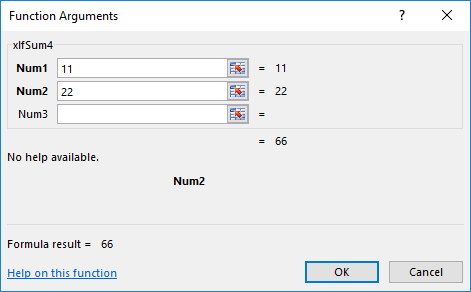Fig 4.1 Function Arguments for xlfSum4 - two compulsory arguments (Num1 and Num2) with bold font, and one optional argument (Num3) with non-bold font. Num3 is blank so the default value, 33 is assigned

## 5. UDF with an unspecified number of arguments (paramarray)

A ParamArray is an array of parameters.

• The array is equivalent to a dynamic array (the ArrayName() has empty brackets) of type Variant
• The ParamArray must be the last argument, and can be the only argument
• A ParamArray argument cannot be used with Optional arguments
• The ParamArray always has base 0 regardless of the Option Base declaration

Code 5: Function procedure xlfSum5 returns sum of 2 non-contiguous WS ranges
Function xlfSum5(ParamArray Num() As Variant) As Double

' Returns the sum of range object
Dim i As Integer
Dim Item As Range

For i = LBound(Num) To UBound(Num)
For Each Item In Num(i)
xlfSum5 = xlfSum5 + Item
Next Item
Next i

End Function

' ================================
Sub Run_xlfSum5()   ' Scroll down to view code
Dim Arr As String
Dim Ans As Double

Arr = "={10,20,30,40;"
Arr = Arr & "55,66,77,88;"
Arr = Arr & "90,100,110,120}"

With ActiveSheet
.Range("C70:F72").FormulaArray = Arr
End With

Ans = xlfSum5(Range("C70:E72"), Range("F70:F72"))

MsgBox "The sum of range with the ParamArray argument equals " & Ans, Title:="xlfSum5 demo"

End Sub


Code 5 by line number:

1. Line 110: Procedure declaration: a Public Function procedure named xlfSum5 with mixed case name. One argument, a ParamArray named Num() that must be of type Variant. In this example, each element of Num() array will store a Range
2. Line 116 to line 121: Is a double For...Next loop that accumulates the values of the array elements
3. Line 116: In the test procedure, two array are passed to Num(), so Num() has lower bound 0, and upper bound 1
4. Line 117: The For...Each procedure loops through each element of the array on a row by row basis
5. Line 119: Accumulates the progressive total (sum) of the elements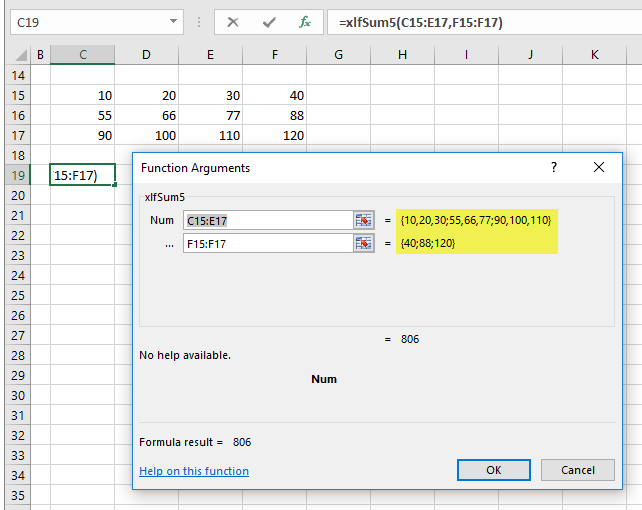Fig 5.1 Locals window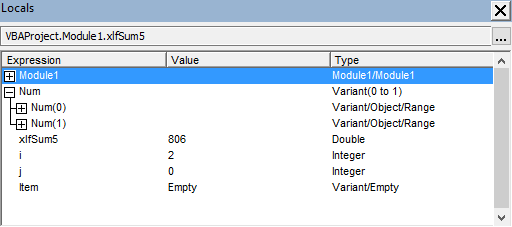Fig 5.2 Locals window with ParamArray Num, a Variant indexed (0 to 1); elements Num(0) and Num(1) each containing a Variant/Range object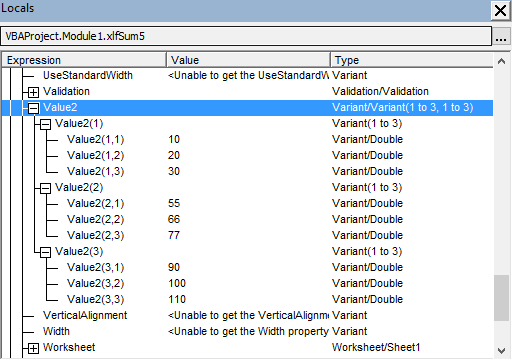Fig 5.3 Locals window with expanded view of Num(0): Variant(1 to 3, 1 to 3)

Reading elements of ParamArray element 0 (shown in figure 5.3):

• Debug.Print Num(0)(2, 3) returns 77
• This means that the Variant array Num() contains an array with values from the range object. These values are identified by the Value2 property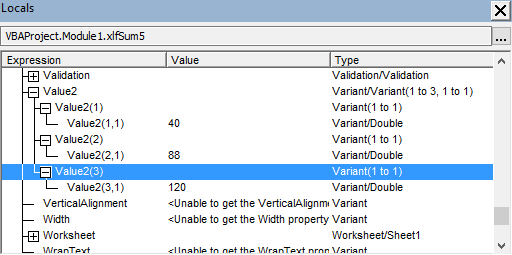Fig 5.4 Locals window with expanded view of Num(1): Variant(1 to 3, 1 to 1)

Reading elements of ParamArray element 1 (shown in figure 5.4):

• Debug.Print Num(1)(2, 1) returns 88
• Debug.Print Num(1)(2) returns 88